# Analyzing Variation with Histograms, KDE, and the Bootstrap

Assume you have a dataset that is a clean sample of a measured variable. It could be a critical dimension of a product, delivery lead times from a supplier, or environmental characteristics like temperature and humidity. How do you make it talk about the variable’s distribution? This post explores this challenge in the simple case of 1-dimensional data. I have used methods from histograms to KDE and the Bootstrap, varying in vintage from the 1890s to the 1980s:

Other methods were surely invented for the same purpose between 1895 and 1960 or since 1979, that I don’t know about or haven’t used. Readers are welcome to point them out.

The ones discussed here are not black boxes, automatically producing answers from a stream of data. All require a human to tune the settings of the tools. And this human needs to know the back story of the data.

## Karl Pearson’s Histograms

One approach, for numeric variables, is to divide their range into bins, throw the individual values into the bins, count the contents of the bins, and plot them in a bar chart, as in this example:Karl Pearson is credited with inventing this technique in 1895, and it is still popular 137 years later. For some purposes, you don’t need to go further. Tony Burns cites the case of a supplier providing parts with a dimension having what appears to be a truncated histogram, raising the question of whether they are meeting specs by weeding out parts. The problems, however, are not always this simple. For example, there is more to studying process capability than plotting a histogram.

## John Tukey’s Stem-and-Leaf Diagrams

One issue with histograms that John Tukey tried to address with his stem-and-leaf plots is the loss of information associated with reducing numerical data to bin counts. In the example above, we go from ~200 actual petal-length measurements to 13 bin counts. In 1977, John Tukey proposed the stem-and-leaf plot. His intent was to show the bin counts graphically while retaining the raw data in the chart.

Like the histogram, the stem-and-leaf plot is taught in American Middle Schools. The only place I have seen it used, however, is in schedule displays at Japanese train stations. The stems are the hours and the leaves the minutes within each hour when the trains arrive :This schedule lets you see both the traffic intensity by the hour and the arrival time of each train by the minute. For data analysis, however, the advantages of the stem-and-leaf plot were not compelling enough for it to unseat the old histogram.

## Limitations of Histograms

In the petal length example, the bins are 0.5\, cm wide, starting at length = 0.5 cm. To generate a histogram, you need to choose a starting point for the first bin and a bin width.

As an example, I’ll use a water quality dataset collected by the Indian government in 2021. Among other variables, it contains min and max measurements of dissolved oxygen at 617 water stations throughout the country, which I converted into midrange and range, and then focused on the midrange.

Dissolved oxygen in water sustains life, so its concentration is a critical characteristic. It won’t be exactly the same in two bodies of water, but the measurements only have two significant digits, causing some to match.

### Effect of shifting the bins

Here are two histograms, identical in every way, except for a .25 mg/l shift in the location of the bin boundaries: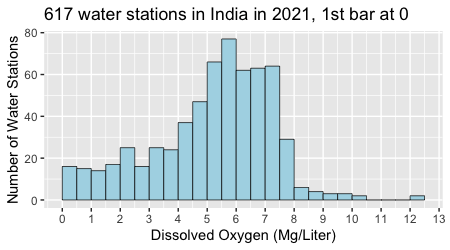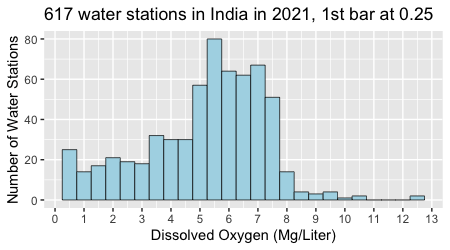If you start the first bin at 0 mg/l, the distribution looks flat at the beginning but, if you start it at 0.25 mg/l instead, it seems to have a minor mode around 0.5 mg/l. Which is it? The histogram won’t tell you.

### Effect of bin width

Following are six histograms of the same data with different bin widths:The narrow bins show a spike near 0 that the wider bins blur. As we shall see, the scale issue does not disappear with KDE but can actually be put to use.

### Gaming opportunities

Histogram bins are a gaming opportunity. If you are an activist who wants lakes full of fish, you will use small bins to raise the alarm about low oxygen levels and push for government action to revitalize the water bodies. If you are an official using the “administrative won’t” to thwart the political will, you will use larger bins to hide the problems.

There are, in fact, few techniques that malicious players cannot game. It does not negate their value to honest analysts trying to make sense of data. It behooves recipients of data products to be wary and inquisitive.

### Histograms and distributions

If the data is a sample of  N independent and identically distributed variables \left( X_j, j = 1,\dots,N\right), with  n_i points falling in the bin bounded by \left[a_i, a_{i+1}\right], then, regardless of the distribution of the points,  n_i is binomial and the law of large numbers tells us that, for any  j,

\lim_{N \to \infty}\frac{n_i}{N} = P\left( a_i \le X_j \lt a_{i+1}\right)

It means that, with a large enough sample, we can estimate the probability of a new variable falling into a particular bin from the relative frequencies observed in the sample. Bin probabilities, however, are not generally what you are after, and it doesn’t work in the tails, where an empty bin with two non-empty neighbors, has a relative frequency of 0 and is therefore assigned a probability of 0 when you know it’s not true. This is similar to the problem of acceptance sampling with low ppm defectives.

### Some conclusions

Throwing data into arbitrary bins and reducing them to a small number of bin counts is crude. There are more gentle and effective ways to make them talk.

## Ranks and Cumulative Distributions

The cumulative frequency plot below is generated directly from the raw data, after ordering them. There are no settings with which to game it. It is a staircase, to which each data point contributes a step of equal height \frac{1}{N} and varying width, determined by the difference in Dissolved Oxygen content at consecutive points. With 617 points, it already looks smooth; with 30,000 points, the individual stairs would be invisible.### What is there to see?

Let’s annotate the interesting features of this chart: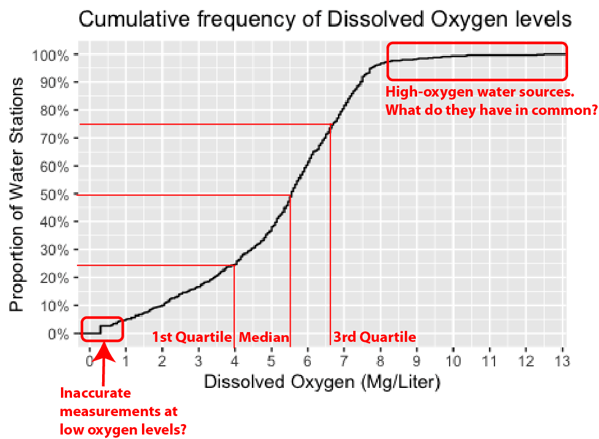Without knowing anything about oxygen in water, what can we say from this chart? Since high levels of dissolved oxygen in water are desirable, the long tail in the upper right-hand corner is of particular interest.

Do these few bodies of water have any common characteristics that you could engineer into the many poorer ones?  At bottom left, the spike 0.3 mg/l raises the question of whether the measurement instruments could accurately capture values  \lt 0.3 mg/l. Then along the way from bottom-left to top-right, you can pick up quartiles and the median.

### Smoothing with Moving Averages and KDE

Although it isn’t necessarily obvious when you read about it, Kernel Density Estimation (KDE) is a moving-average technique. Before getting to it, it helps to go through some basics of smoothing with moving averages.

#### Moving average of a sequence of data

Among the many methods to smooth out noise is the moving average, commonly used with time series in economics. If the purpose is forecasting a time series like daily sales, then you use only past values.

To bring to light a trend that is obscured by fluctuations in space series like natural radioactivity at various depths in an oil well, you use values on both sides of the point. Rob Hyndman shows this example of moving averages of yearly electricity sales in South Australia, at various levels:This example shows how, as you extend the range of the average from 3 to 9 years, the resulting curve grows flatter, and zones extend at the beginning and the end where you don’t have enough data to calculate the moving average.

It’s a simple calculation. If, at the nth point, you include k points before and after, the simple, two-sided moving average of a series of data points y_i is

m_n = \frac{y_{n-k} +\dots + y_{n+k}}{2k+1}= m_{n-1} -\frac{y_{n-k-1}}{2k+1} + \frac{y_{n+k}}{2k+1}

As is clear from this equation, even if the y_i are i.i.d., the m_i are not, as two consecutive values share all but two of their terms.

The weighted moving average is a refinement of this approach that gives different weights w_i to the points before and after depending on how far they are:

m_n = \frac{w_{-k}y_{n-k} +\dots + w_ky_{n+k}}{2k+1}= m_{n-1} -\frac{w_{-k}y_{n-k-1}}{2k+1} + \frac{w_ky_{n+k}}{2k+1}

#### Moving averages of measurements along a continuous axis

As they have not been taken over time at the same locations, our dissolved oxygen readings from water bodies in India are not a time series. As we don’t have the geographical coordinates of the water bodies, they are not a space series either. Time series usually have points collected at fixed intervals, whether it’s once a year or every second.

A manufacturing process in one-piece flow puts out one unit every takt during production, but the interval between consecutive units is different when the line stops for a break or a shift. If a process makes parts in batches, they don’t come out individually at fixed intervals, and the time or distance between consecutive points matters.

Rather than just a sequence of y_i, the more general pattern is to have a sequence \left (x_i, y_i \right ), where the lags   x_{i+1}-x_i vary and the dataset is viewed as a sample drawn from a continuum of values of  x.

In this case, the simple moving average is the ratio of the area under the curve  y for the interval \left [ x-s, x+s \right ], divided the length 2s of the interval.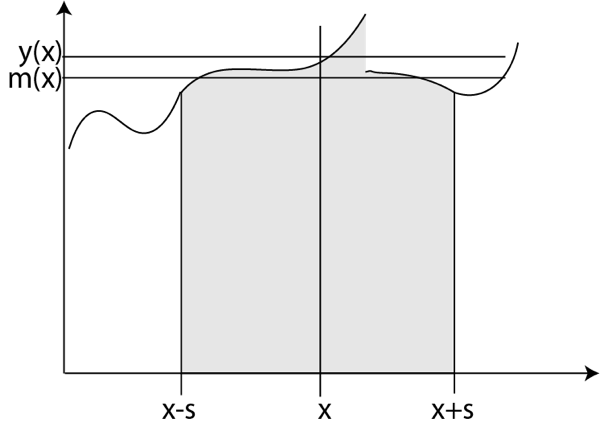The formula for this simple moving average is

m\left ( x \right )= \frac{1}{2s}\int_{x-s}^{x+s}y\left ( u \right )du

#### Weights and Kernels

If we introduce the weight function w(u) = \frac{1}{2s}I_{\left [ -s,s \right ]}\left ( u \right ) = \frac{1}{s}\times\frac{1}{2}I_{\left [ -1,1 \right ]}\left ( \frac{u}{s} \right ) that is worth \frac{1}{2s} between -s and +s and 0 everywhere else, we can rewrite this formula as

m\left ( x \right )= \int_{-\infty}^{+\infty}y\left ( u \right )w(x-u)du

which is known at the convolution of y with the weight function w, which we call a kernel. It’s positive, symmetric around 0, its area under the curve is 1, and it has a scale parameter s that can be adjusted for more or less smoothing. This kernel is, in fact, the probability distribution function of the uniform distribution in \left [ -s,s \right ]. It gives equal weight to all points in this interval. As in the discrete case, different kernels can give more weight to points that are nearer rather than farther. The general form of a scaled kernel is w_s(u) = \frac{1}{s}\times K\left ( \frac{u}{s} \right ), with K symmetric, positive and summing up to 1. Here are a few options:#### What’s special about the Gaussian kernel

As you increase the kernel’s scale, or bandwidth, you expect peaks to erode and valleys to fill up as in this picture, but only the Gaussian kernel guarantees this.Among the possible kernels, the Gaussian, with  \sigma as a scale, has the unique property that, when you increase \sigma, the peaks in the smoothed signal always go down, and the valleys always go up. At least it’s true for first-order extrema, characterized by a zero derivative and non-zero curvature. Jean Babaud’s proof of this result was published in a 1986 paper that I co-wrote.

In addition, smoothing with a Gaussian kernel also has a physical interpretation in diffusion and heat transfer by conduction. The profile of concentrations or temperature at time t is the convolution of the profile at time  0 with a Gaussian kernel having a standard deviation proportional to  \sqrt{t}. It’s the general solution to the equation of diffusion with a known initial condition.

### Kernel Density Estimation (KDE)

For a random variable, the probability distribution function (pdf) is the derivative of the cumulative distribution function (cdf). For our sample of dissolved oxygen data in water bodies in India, the cumulative relative frequency function is a staircase, and its derivative is 0 everywhere except at the x_i, where it is a Dirac impulse, shown below as an up-arrow.Convolution with a kernel is not, in general, simple, but convolving with a Dirac impulse is. By definition of the Dirac impulse \delta, at x_i with a kernel w, it just gives you a copy of the kernel centered on x_i :

\int_{-\infty}^{+\infty}\delta\left (u -x_i\right )w(x-u)du = w\left ( x - x_i \right )

Formally, the cumulative relative frequency function is

G\left ( x \right )= \frac{1}{N}\sum_{i=1}^{N}I(x-x_i)

where  I(x)=0 for  x < 0 and I(x)=1  for  x \ge 0 . It’s the Heaviside function, and  G is the staircase we have seen. It’s derivative is the sample distribution

g\left ( x \right )= \frac{1}{N}\sum_{i=1}^{N}\delta(x-x_i)

By linearity, when we smooth it by convolving it with the kernel w, we get the kernel density estimator (KDE) \hat{f} with the kernel w for the probability density function f of the common distribution of the  X_i. It is simply

\hat{f}\left ( x \right )= \frac{1}{N}\sum_{i=1}^{N}w(x-x_i)

First, you place a copy of the kernel at each point  x_i in the sample and, at each x. Then you take the average of the values at x of all these shifted kernels. This equation is where the Wikipedia article on KDE starts. It tells us that the technique was invented by Emanuel Parzen and Murray Rosenblatt in the 1950s but does not explain the connection with moving averages. It is a moving average, not of the data points themselves, but of the sum of Dirac impulses that represents their density on the x-axis.

### KDE with a default scale

Let’s look at what KDE with Gaussian kernels tells us about dissolved oxygen in Indian water bodies, using the default scale of the density function in R.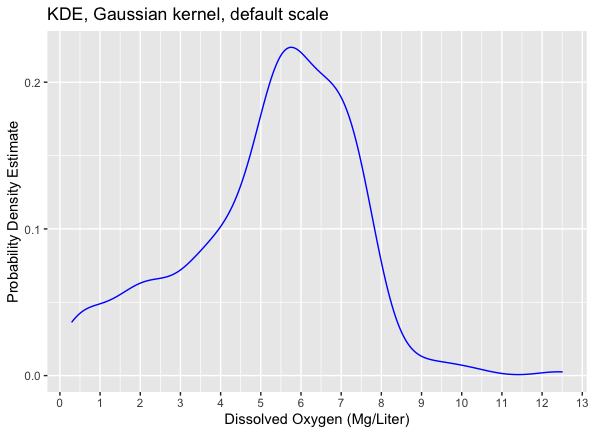How well does it do in smoothing away sample-specific asperities while visualizing the underlying distribution? The first thing we can do is compare its cumulative values with the staircase cumulative frequencies from the data: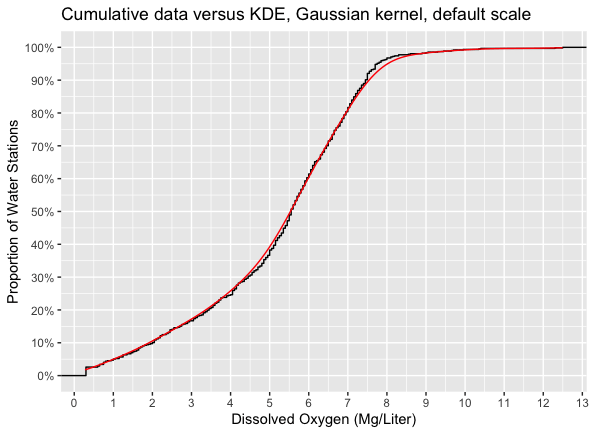While not perfect, it’s pretty close for a calculation that is nearly instant on a current laptop business computer and requires a single instruction.

The default scale s used in R is the empirical Silverman’s rule of thumb:

s=0.9\times\min \left({\hat {\sigma }},{\frac {Q}{1.34}}\right)\,N^{-{\frac {1}{5}}}

where \hat {\sigma } is the standard deviation estimate from the data and Q the interquartile distance. In our dissolved oxygen dataset, the default scale, also known as bandwidth, is s=0.492.

### KDE with multiple scales

Like histogram bins and locations, KDE ranges are a gaming opportunity. In KDE, rather than a default scale, we can use a range of scales on a ridgeline plot, similar to lines in a mountain landscape:

#### Edge Problems

Besides the bump for low values that shows with the narrowest ranges, the ridgeline plot also shows positive densities for negative concentrations of dissolved oxygen, which make no sense. The kernel doesn’t know that concentrations cannot be negative. This variable actually has an upper bound too. Under the conditions at the earth’s surface, there cannot be more than 18 mg/l of dissolved oxygen in water.

This upper bound, however, is far from the maximum of the data. Many major characteristics are also bounded, including temperature, pressure, or weight, but bounds play no role if the readings are far enough from them. Unless you are working in cold physics, temperatures are far enough from absolute zero to treat their range as unbounded.

There are tricks to applying KDE for variables that are bounded on one or both sides, involving either using a different kernel or transforming the data. For the purpose of exploring this data, given that the low values bump is suspicious, it’s OK to ignore the spurious tails. On the other hand, the plots for \sigma = 0.1,\dots,0.5 show three valleys gradually eroding that suggest that the dataset is heterogeneous — that is, it may commingle data subsets from several distributions.

#### Opportunities to Slice and Dice the Dataset

The dataset contains several classification keys:

• Water body types — lake, pond, tank, wetland.
• Indian states — Andhra Pradesh, Assam, Bihar, Chandigarh, Chhattisgarh, Delhi, Goa, Gujarat, Haryana, Himachal Pradesh,  Jammu & Kashmir, Jharkhand, Karnataka, Kerala, Lakshadweep, Madhya Pradesh, Manipur, Meghalaya, Mizoram, Nagaland, Odisha, Puducherry, Punjab, Rajasthan, Tamil Nadu, Telangana, Tripura, Uttar Pradesh, Uttarakhand, West Bengal
• Codes and names from monitoring locations, with which you could look up geographical coordinates.

The red lines in the plot prompt the analyst to slice and dice the data in search of homogeneous subsets. It’s not a foregone conclusion that the data come from independent and identically distributed (i.i.d.) variables. Water bodies in the same river basin, for example, could have correlated characteristics, and there could be a latitude trend.

The dataset contains points from the states of Kerala and Haryana, 2,500 km to the North. Any serious effort to fit a model to these data would have to start with analyzing whether latitude makes a difference. This is the backstory of the data. This post is not such an effort. We are only trying to explain tools and, for this purpose, we can pretend the data is a sample of i.i.d. variables, and use it to infer this distribution.

## Fitting a Model

What if we want to generalize from what we learned on this sample of 617 bodies of water, and make statements about other bodies of water? To the Canadian government, the lowest acceptable dissolved oxygen concentration in warm water systems is 6 mg/l for aquatic life in early life stages and 5.5 in later stages. The requirements are higher in cold water, 9.5 mg/l for early stages and 6.5 in later stages. The median of the India water dataset in 5.55 mg/l. Can we conclude that half the water bodies in India are below Canadian specs for sustaining aquatic life? The next question would be whether water bodies below the Canadian spec actually support aquatic life.

### Objective: Filtering Sample-Specific Details

A model of all water bodies in India based on this sample would have to incorporate everything about the sample that is generic, but nothing would likely be different from another sample.  The long tail should be part of the model, but the exact location of each step in the staircase is specific to the sample. Another sample of 617 water bodies would have a cumulative frequency curve with the same shape when viewed from a distance, but a closeup look would reveal steps at different locations.Traditionally, probability theory has been focused on a small menagerie of mathematically tractable distributions: Gaussian (a.k.a. Normal), Binomial, Poisson, Lognormal, Gamma, Beta, Weibull, Dirac, Uniform, etc. In Converting Capabilities, Don Wheeler only considers fitting four distributions — Gaussian, Lorgnormal, stretched Gamma, and Weibull —  to a histogram of fractions defective in 250 samples, in order to conclude that the error bars overlap so much that the choice of one model makes no material difference.

With his razor, Occam would conclude that you should use the simplest model. Wheeler, instead, concludes that fitting a model serves no useful purpose and that you should stick with empirical estimates.

#### What are “empirical estimates”?

“Empirical estimates” result from applying some formula to the data, like the ratio of counts of defectives to sample size.  The meaning of such ratios hinges on assumptions about the data, in other words, on some model. This particular one is an estimation of the probability p that any given unit within a sample is defective if the defects occur independently.

#### The Traditional Set of Distributions

There were several reasons for the attention given to the traditional set of distributions:

1. They were mathematically tractable by human computers with slide rules and books of tables, and have been studied extensively for decades when not centuries.
2. There are cases where reasonable assumptions about the data lead to these models. E.T. Jaynes cites astronomer John Herschel’s derivation of the Gaussian as a model for errors in the measurement of star positions. Herschel assumed that errors in latitude and longitude are independent and that their magnitudes have the same distribution in every direction. This was enough for the math to exclude every distribution but the Gaussian.
3. There are other cases where these models work for aggregates of data, even when they don’t for the raw data. The independent choices of millions of consumers regarding a product add up to a Gaussian sales volume. If a system fails when any one of its many independent components does, the failures of the system can be modeled as a Poisson process. This holds even when the component processes are not Poisson. Medians, quantiles, or extreme values can often be modeled in ways that are largely independent of the underlying distributions.  They are called “distribution-free.”

These models, however, are sometimes viciously misapplied. In business, for example, individual performance reviews are twisted to produce gaussian distributions in groups by instructing managers to “grade on a curve.”

### Fitting a Model Today

Our goal is not to have a mathematical formula. Instead, we want a curve that retains the distribution information in the data while smoothing over details that would be different in another sample from the same distribution. Show too many details, like the top curve for \sigma = 0.1 in the ridgeline plot and you are overfitting. Show too few, as in the bottom curve for \sigma = 1, and you miss key features. It is for the analyst to choose the goldilocks bandwidth to achieve the goal. An unscrupulous analyst could use bandwidths like histogram bins to game the results. A ridgeline plot, however, would expose the ploy.

#### The KDE as a Model

KDE generates distributions that are specific to data sets. We don’t have to fit a model from our catalog of distributions to the data. We can choose to work directly with the KDE. Of course, it comes with its own challenges. Besides a curve on a chart, it comes in the form of a lookup table. By default, the density function in R gives you 512 values. You can set this number to any higher power of 2: 1024, 2048, 4096, etc.

Assuming the analyst finds that the low-value readings are indeed flawed but fails to find any meaningful clustering of data to account for the red lines in the ridgeline plot, he or she may trim off the values under 0.5 mg/l and choose  \sigma = 0.5, resulting in the following curve:#### Using Bootstrapping to Check the KDE Model

The KDE is ugly but it can be used to set confidence intervals or thresholds for statistical tests. It also begs the question of what the same curve would look like if drawn from other samples. Strictly speaking, answering it would require taking measurements from multiple samples of >600 bodies of water in India.

Short of doing this, we can bootstrap alternative samples from the actual one. This is a procedure invented by Bradley Efron in 1979, which consists of throwing all the data points in an urn and drawing them one by one with replacement until you reach the number in the original set.

The probability of drawing a point within a range is the relative frequency of points within that range. Based on the law of large numbers, it estimates the underlying probability of this range. The bootstrap samples differ from the original sample because of drawing with replacement. It leads to never drawing some points while drawing others more than once.

A common use of this method is to calculate a complex statistic on, say, 1,000 bootstrap samples.  You use the average of the statistic on all these samples as an estimate. Then you use its standard deviation as standard error. Here, we can plot the KDEs for 5 bootstrap samples in different colors and compare it to the KDE for the actual sample, in black: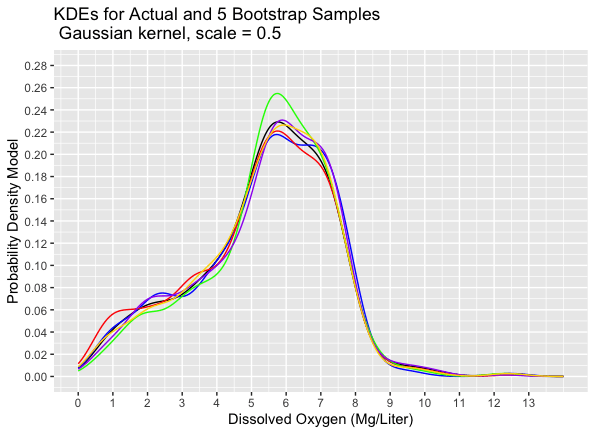We could then measure the distances between curves in various ways:

• The maximum absolute value of their differences
• The area between the curves
• The root-mean-square of their differences

Strong opinions abound about the best way to do this, and it is beyond the scope of this post.

## Conclusion

If you limit yourself to using methods that predate the computer, you are a data dinosaur.  Data mammals will outperform you. If you dismiss all new developments as fancy math and discourage others from learning, you are a data Luddite. Be neither a dinosaur nor a Luddite! Study the state of the art in data science, and you will find some of it useful.